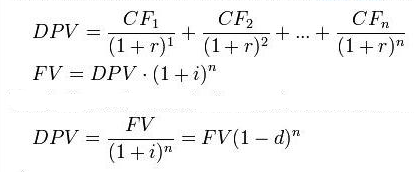## Tuesday, April 3, 2012

### Equity Market Fair Value (Morningstar)

A relatively accurate fair value could give the trader a feel for whether an asset's current bid/offer is respectively high/low. Valuation of general equity markets, or particular sectors, would intuitively take a truckload of number crunching, luckily it is available at Morningstar.

About the Morningstar Fair Value Calculations
source: How Fair Value and Target Price Differ
"
To derive the fair value estimates, we use our proprietary discounted cash-flow (DCF) model. This model assumes that the stock's value is equal to the total of the free cash flows the company is expected to generate in the future, discounted back to the present. So, the first step is to project how much cash a firm is likely to produce over a number of years, and subtract the amount needed for capital improvements and increases in working capital to keep the business growing. Whatever profits are left over belong to the shareholders. The second step is to discount those profits to understand how much they are worth today.

As with any DCF model, the ending value is highly sensitive to the analyst's projections of future top- and bottom-line growth. In addition, the cost of capital, which is determined by the firm's capital structure and its riskiness, is another influential factor in the fair value estimate. (For more discussion of the nuts and bolts of Morningstar's DCF model, please see David Kathman's article "How Morningstar Values Stocks".)

source: Wikipedia on DCF
"
The discounted cash flow formula is derived from the future value formula for calculating the time value of money and compounding returns.where
• DPV is the discounted present value of the future cash flow (FV), or FV adjusted for the delay in receipt;
•
• FV is the nominal value of a cash flow amount in a future period;
•
• i is the interest rate, which reflects the cost of tying up capital and may also allow for the risk that the payment may not be received in full;
•
• d is the discount rate, which is i/(1+i), i.e. the interest rate expressed as a deduction at the beginning of the year instead of an addition at the end of the year;
•
• n is the time in years before the future cash flow occurs.
"